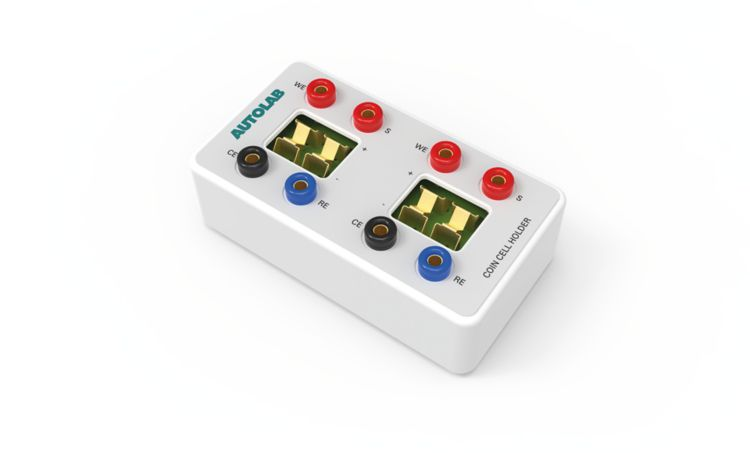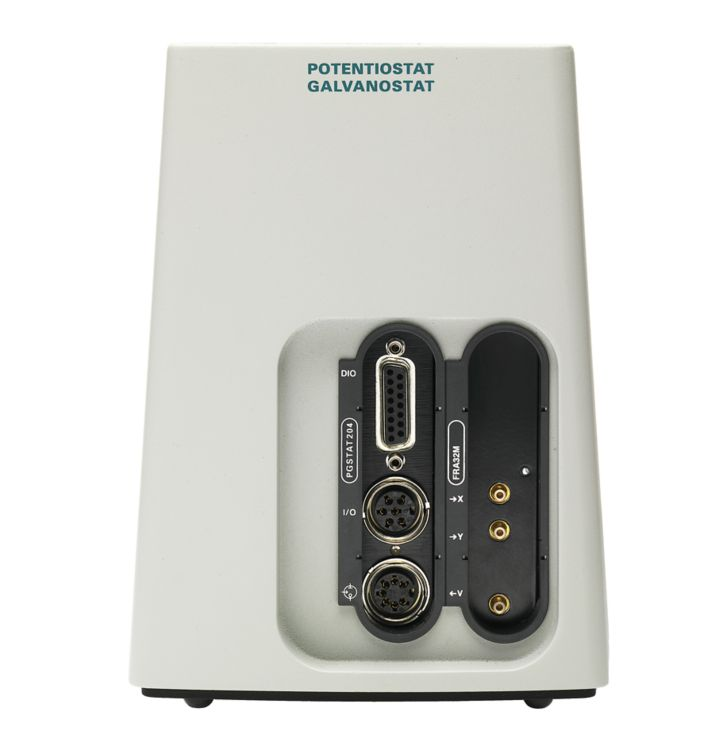Figure 1. The Metrohm Autolab DuoCoin Cell Holder

The Metrohm Autolab DuoCoin Cell Holder, shown in Figure 1, has been developed to perform electrochemical experiments on coin cell batteries.

The DuoCoin Cell Holder can host up to two coin cells, each of 3.2 mm maximum thickness and 24 mm maximum diameter. Typical coin cell sizes which can be hosted in the DuoCoin Cell Holder are CR2016, CR2020, CR2025, CR2032, CR2325, and CR2330. Each connector of the DuoCoin Cell Holder is directly connected to the battery. Therefore, the leads sensing the potential are separated from the leads carrying the current, resulting in a minimized voltage drop due to the impedance of the wires.

In this application note, electrochemical impedance spectroscopy (EIS) is used to test a commercial battery. As comparison, the results from the four-electrode configuration are compared with results from two-electrode configuration, in which the RE and CE leads are connected together, as well as the WE and S leads.

The difference in how the leads are connected results in different measured impedance values.Figure 2. The Metrohm Autolab PGSTAT204, equipped with the FRA32M module.

For the EIS measurements, a Metrohm Autolab PGSTAT204 equipped with a FRA32M module is used (Figure 2).

The battery used for the experiments is a rechargeable Li-ion, Panasonic VL2330, with 30 mAh of nominal capacity and a nominal voltage of 3 V.

EIS potentiostatic measurements are performed at open circuit potential (OCP), between 10 kHz and 100 mHz, 10 mV amplitude, with a rate of 10 frequencies per decade.

In Figure 3 the Nyquist plot of measurement performed with the four-electrode configuration (red dots) are compared with the results obtained with two-electrode configuration (blue and red dots).

Regarding the two-electrode configuration, EIS measurement is performed with connecting the WE and S leads together and the CE and RE leads together, having therefore the RE and S leads closer to the battery (blue dots). Another measurement has been carried out with the leads connections inverted, so connecting S and WE leads together and the RE and CE together, having the WE and CE leads closer to the battery (green dots).

Figure 3. Nyquist plots from EIS measurements performed on the Li-ion battery with four-terminal (red dots) and two-terminal (red dots) sensing configurations.

While there is no appreciable difference between the two-lead configurations, the Nyquist plot corresponding to the four-terminal configuration is shifted towards lower impedance values, with respect to the Nyquist plots resulting from the two-terminal configuration. In Figure 4, the magnification at high frequencies of Figure 3 shows a difference in impedance of approximately 170 mΩ.

Figure 4. Magnification of Figure 3 at high frequencies.

However, the difference is more evident at low frequencies, as shown in Figure 5, where the difference in impedance between the four-terminal and two-terminal configuration at the end of the semicircle is approximately 2 Ω.

Figure 5. Magnification of Figure 3 at low frequencies.

Finally, it is worth noting that the use of the four-terminal sensing configuration is important only when low-impedance devices are under investigation, like batteries, since the contribution of the wires to the overall impedance is low.

The DuoCoin Cell Holder is introduced. EIS measurements on a commercial coin cell battery are performed. Differences in impedance between the four-terminal configuration and two-terminal configuration is highlighted, putting in evidence the importance of having a direct four-terminal configuration, when low-impedance DUTs are investigated.